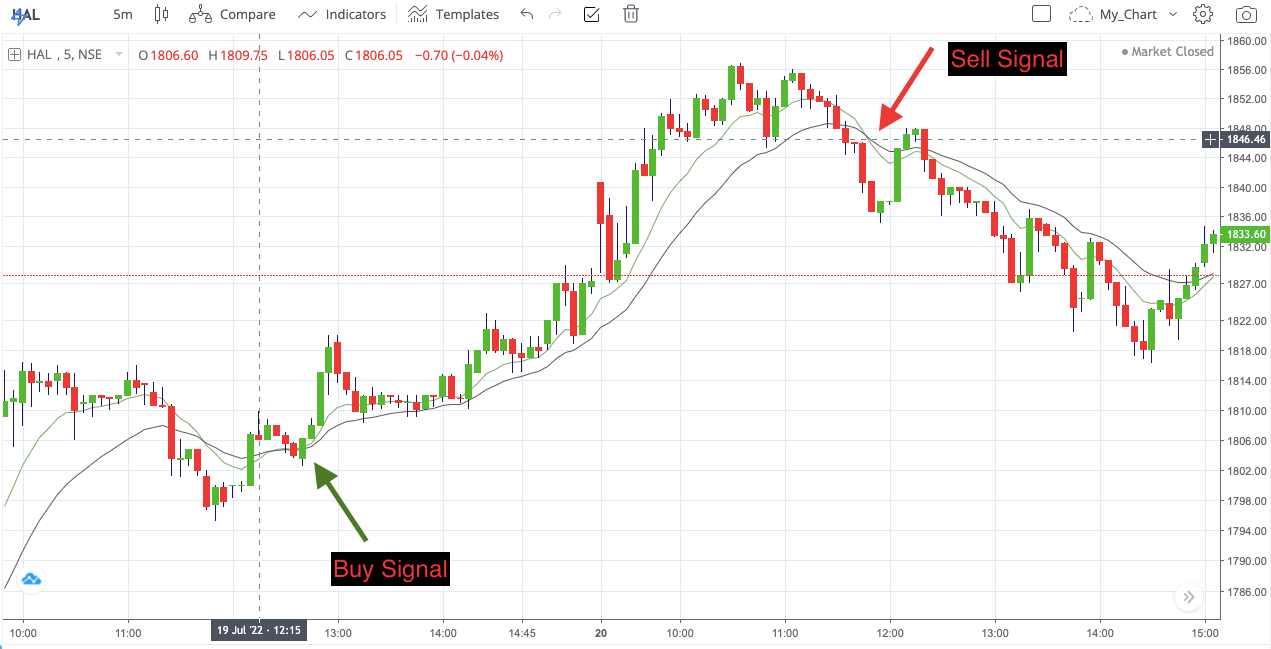Select Page

## Best Moving Average Strategy for Trading & Investing

There are many moving average strategies across the market. Some of them work best and some of them do not work.

Today I am going to discuss two moving average strategies that work best in intraday trading, swing or positional trading, and investing also.

So, don’t go anywhere else. Take a cup of coffee and sit tight.

Let’s start.

## Moving Average Strategy-1

In this strategy, you may use two moving averages.

One is a shorter period, and another is a longer period moving average.

i) Buy when the shorter period moving average crosses above the longer period moving average. It is called bullish crossover.

ii) Sell when the shorter period moving average crosses below the longer period moving average. It is called a bearish crossover.Buy when green color shorter period moving average crosses above black color longer period moving average.
And sell when the shorter period moving average crosses below longer period moving averages.
Here the shorter period EMA is 10 EMA and the longer one is 20 EMA.

iii) You should add RSI to get a confirmed buy and sell signal.

iv) Bullish crossover is called Golden Cross whereas bearish crossover is called Death Cross.

The commonly used moving averages are 50, 100, and 200-period moving averages.

And for trading short-term trends you may use a set of 10 and 20-period moving averages.

## Moving Average Strategy- 2 (triple moving average crossover):

in this strategy, you may use three moving averages- short-term, mid-term, and long-term moving average.

The short-term moving average is used to get a buy or sell signal.

When the short-term moving average crosses above the mid-term moving average it gives a buy signal and when it crosses below the mid-term moving average it gives a sell signal.

So, the short-term and mid-term moving averages are used to get entry and exit signals.

And the long-term moving average is used to get information about the trend.

So, you may buy when the short-term moving average crosses above both the mid-term and long-term moving average.

And exit when the short-term moving average crosses down the mid-term moving average.Sell when the short-term moving average crosses down both the mid-term and long-term moving average.

And exit when the short-term moving average crosses above the mid-term moving average.

And remember each moving average should be multiple of two from one another.

You may use both the above moving average strategies for positional, intraday and long-term perspectives.

Now let’s see how you may use these strategies.

## Moving Average Strategy for Positional Trading:

For positional trading, you may use 14, 21, and 50-period EMA.

In a trending market, you may go long(buy) when 14 period EMA crosses above both 21-period and 50-period moving averages.

And you may go short (sell first) when 14-period EMA crosses below both 21-period EMA and 50-period EMA.

Exit when 14 period EMA crosses above 21 periods EMA.

If you think about the timeframe, then you may use 1-hour chart to enter and exit a trade in positional trading.

## Best Moving Average Settings for Day Trading:

In an uptrend, go long (buy first) when 5-period EMA crosses above both 10-period and 20-period EMA and exit when 5-period EMA crosses below 10-period EMA.

In a downtrend, go short( sell first) when 5-period EMA crosses below both 10-period and 20-period EMA.

And exit when 5-period EMA crosses above 10-period EMA.

## Conclusion:

Since the moving average strategy works best in a trending market, your work should first identify the market situation.

If the market is trending then go with these moving average strategies.

Otherwise, in a sideways market condition, you may face several losses as in a range-bound market price frequently crosses above and below moving averages.

So, you should avoid these strategies in a choppy or sideways market.

Also, you may use other indicators like RSI and volume to confirm your trading entry and exit.